• 快速傅里叶变换 (fast Fourier transform),即利用计算机计算离散傅里叶变换（DFT)的高效、快速计算方法的统称，简称FFT。快速傅里叶变换是1965年由J.W.库利和T.W.图基提出的。采用这种算法能使计算机计算离散傅里叶...
FFT前言

快速傅里叶变换 (fast Fourier transform),即利用计算机计算离散傅里叶变换（DFT)的高效、快速计算方法的统称，简称FFT。快速傅里叶变换是1965年由J.W.库利和T.W.图基提出的。采用这种算法能使计算机计算离散傅里叶变换所需要的乘法次数大为减少，特别是被变换的抽样点数N越多，FFT算法计算量的节省就越显著。
FFT（Fast Fourier Transformation） 是离散傅氏变换（DFT）的快速算法。即为快速傅氏变换。它是根据离散傅氏变换的奇、偶、虚、实等特性，对离散傅立叶变换的算法进行改进获得的。
——百度百科

FFT（Fast Fourier Transformation），中文名快速傅里叶变换，用来加速多项式乘法
朴素高精度乘法时间$O(n^2)$，但$FFT$能$O(n\log_2 n)$的时间解决
$FFT$名字逼格高，也难懂，其他教程写得让人看不太懂，于是自己随便写一下

建议对复数、三角函数相关知识有所耳闻 （不会也无所谓）

下面难懂的点我会从网上盗

多项式的系数表示法和点值表示法

$FFT$其实是一个用$O(n\log_2n)$的时间将一个用系数表示的多项式转换成它的点值表示的算法

多项式的系数表示和点值表示可以互相转换

系数表示法

一个n-1次n项多项式$f(x)$可以表示为$f(x)=\sum^{n-1}_{i=0}a_ix^i$
也可以用每一项的系数来表示$f(x)$，即$f(x)=\{a_0,a_1,a_2,...,a_{n-1} \}$
这就是系数表示法，也就是平时数学课上用的方法

点值表示法

把多项式放到平面直角坐标系里面，看成一个函数

把$n$个不同的$x$代入，会得出$n$个不同的$y$，在坐标系内就是$n$个不同的点

那么这$n$个点唯一确定该多项式，也就是有且仅有一个多项式满足$∀k,f(x_k)=y_k$

理由很简单，把$n$条式子联立起来成为一个有n条方程的n元方程组，每一项的系数都可以解出来

那么$f(x)$还可以用$f(x)=\{(x_0,f(x_0)),(x_1,f(x_1)),(x_2,f(x_2)),...,(x_{n-1},f(x_{n-1}))\}$来表示
这就是点值表示法

高精度乘法下两种多项式表示法的区别
对于两个用系数表示的多项式，我们把它们相乘
设两个多项式分别为$A(x),B(x)$
我们要枚举$A$每一位的系数与$B$每一位的系数相乘
那么系数表示法做多项式乘法时间复杂度$O(n^2)$
但两个用点值表示的多项式相乘，只需要$O(n)$的时间

什么意思呢？

设两个点值多项式分别为$f(x)=\{(x_0,f(x_0)),(x_1,f(x_1)),(x_2,f(x_2)),...,(x_{n-1},f(x_{n-1}))\}$$g(x)=\{(x_0,g(x_0)),(x_1,g(x_1)),(x_2,g(x_2)),...,(x_{n-1},g(x_{n-1}))\}$
设它们的乘积是$h(x)$，那么$h(x)=\{(x_0,f(x_0)·g(x_0)),(x_1,f(x_1)·g(x_1)),...,(x_{n-1},f(x_{n-1})·g(x_{n-1}))\}$
所以这里的时间复杂度只有一个枚举的$O(n)$

突然感觉高精度乘法能$O(n)$暴艹一堆题？

但是朴素的系数表示法转点值表示法的算法还是$O(n^2)$的，逆操作类似

朴素系数转点值的算法叫DFT（离散傅里叶变换），点值转系数叫IDFT（离散傅里叶逆变换）

难道高精度乘法只能$O(n^2)$了吗？

DFT前置知识&技能

复数

毕竟高中有所以不多说

我们把形如a+bi（a,b均为实数）的数称为复数，其中a称为实部，b称为虚部，i称为虚数单位。当虚部等于零时，这个复数可以视为实数；当z的虚部不等于零时，实部等于零时，常称z为纯虚数。复数域是实数域的代数闭包，也即任何复系数多项式在复数域中总有根。 复数是由意大利米兰学者卡当在十六世纪首次引入，经过达朗贝尔、棣莫弗、欧拉、高斯等人的工作，此概念逐渐为数学家所接受。
——百度百科

初中数学老师会告诉你没有$\sqrt{-1}$，但仅限$R$
扩展至复数集$C$，定义$i^2=-1$，一个复数$z$可以表示为$z=a+bi(a,b\in R)$
其中$a$称为实部，$b$称为虚部，$i$称为虚数单位

在复数集中就可以用$i$表示负数的平方根，如$\sqrt{-7}=\sqrt{7}i$

还可以把复数看成复平面直角坐标系上的一个点，比如下面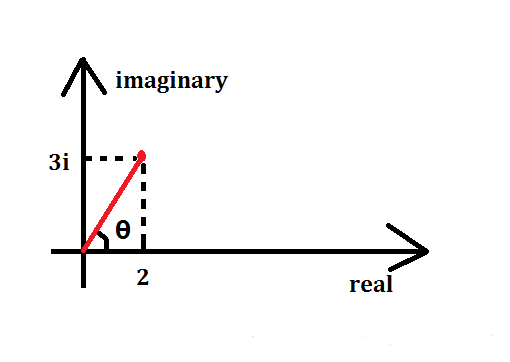$x$轴就是实数集中的坐标轴，$y$轴就是虚数单位$i$轴

这个点$(2,3)$表示的复数就是$2+3i$，或者想象它代表的向量为$(2,3)$
其实我们还可以自己想象 （其实没有这种表达方式） 它可以表示为$(\sqrt{13},\theta)$
一个复数$z$的模定义为它到原点的距离，记为$|z|=\sqrt{a^2+b^2}$
一个复数$z=a+bi$的共轭复数为$a-bi$（虚部取反），记为$\overline{z}=a-bi$

复数的运算

复数不像点或向量，它和实数一样可以进行四则运算
设两个复数分别为$z_1=a+bi,z_2=c+di$，那么
$z_1+z_2=(a+c)+(b+d)i$$z_1z_2=(ac−bd)+(ad+bc)i$
复数相加也满足平行四边形法则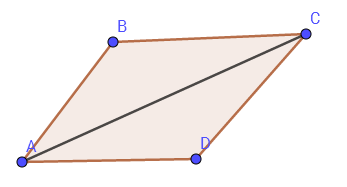这张是从网上盗的

即$AB+AD=AC$
复数相乘还有一个值得注意的小性质
$(a_1,\theta_1)*(a_2,\theta_2)=(a_1a_2,\theta_1+\theta_2)$
即模长相乘，极角相加

DFT（离散傅里叶变换）

一定注意从这里开始所有的$n$都默认为$2$的整数次幂

对于任意系数多项式转点值，当然可以随便取任意$n$个$x$值代入计算
但是暴力计算$x_k^0,x_k^1,...,x_k^{n-1}(k\in[0,n))$当然是$O(n^2)$的时间
其实可以代入一组神奇的$x$，代入以后不用做那么多的次方运算
这些$x$当然不是乱取的，而且取这些$x$值应该就是 傅里叶 的主意了
考虑一下，如果我们代入一些$x$，使每个$x$的若干次方等于$1$，我们就不用做全部的次方运算了
$±1$是可以的，考虑虚数的话$±i$也可以，但只有这四个数远远不够

傅里叶说：这个圆圈上面的点都可以做到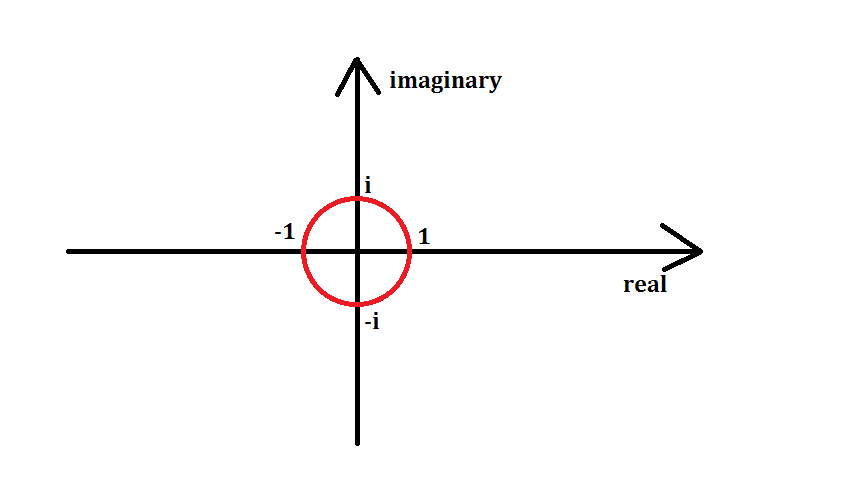以原点为圆心，画一个半径为$1$的单位圆
那么单位圆上所有的点都可以经过若干次次方得到$1$
傅里叶说还要把它给$n$等分了，比如此时$n=8$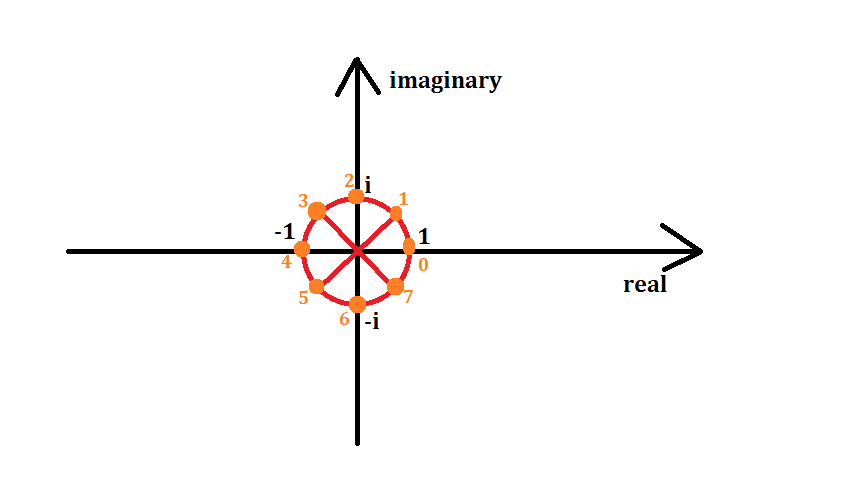橙色点即为$n=8$时要取的点，从$(1,0)$点开始，逆时针从$0$号开始标号，标到$7$号
记编号为$k$的点代表的复数值为$\omega_n^k$，那么由模长相乘，极角相加可知$(\omega_n^1)^k=\omega_n^k$
其中$\omega_n^1$称为$n$次单位根，而且每一个$\omega$都可以求出 （我三角函数不好）
$\omega_n^k=\cos{k\over n}2π+i\sin{k\over n} 2π$
或者说也可以这样解释这条式子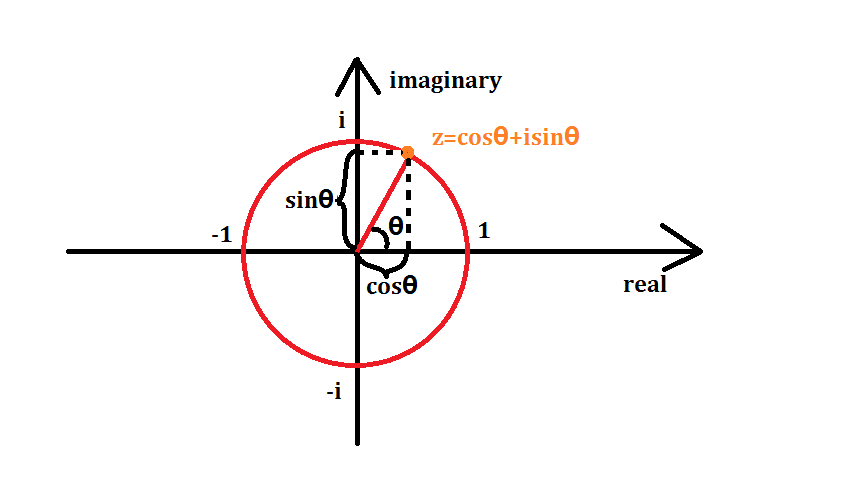注意$sin^2\theta+cos^2\theta=1$什么的，就容易理解了

那么$\omega^0_n,\omega^1_n,...,\omega^{n-1}_n$即为我们要代入的$x_0,x_1,...,x_{n-1}$

单位根的一些性质
推$FFT$的过程中需要用到$\omega$的一些性质

$\omega^k_n=\omega^{2k}_{2n}$

它们表示的点（或向量）表示的复数是相同的

证明

$\omega^k_n=cos{k\over n}2π+isin{k\over n} 2π=cos{2k\over 2n}2π+isin{2k\over 2n} 2π=\omega^{2k}_{2n}$

$\omega^{k+{n \over 2}}_n=-\omega_n^k$

它们表示的点关于原点对称，所表示的复数实部相反，所表示的向量等大反向

证明

$\omega^{n\over 2}_n=cos{{n\over 2}\over n}2\pi+isin{{n\over 2}\over n}2\pi=cos\pi+isin\pi=-1$

（这个东西和$e^{ix}=cosx+isinx$与$e^{i\pi}+1=0$有点关系，我不会就不讲了）

$\omega^0_n=\omega^n_n$

都等于$1$，或$1+0i$

FFT（快速傅里叶变换）
虽然$DFT$搞出来一堆很牛逼的$\omega$作为代入多项式的$x$值
但只是代入这类特殊$x$值法的变换叫做$DFT$而已，还是要代入单位根暴力计算

DFT还是暴力$O(n^2)$…

但$DFT$可以分治来做，于是 FFT（快速傅里叶变换） 就出来了
首先设一个多项式$A(x)$
$A(x)=\sum^{n-1}_{i=0}a_ix^i=a_0+a_1x+a_2x^2+...+a_{n-1}x^{n-1}$
按$A(x)$下标的奇偶性把$A(x)$分成两半，右边再提一个$x$
$A(x)=(a_0+a_2x^2+...+a_{n-2}x^{n-2})+(a_1x+a_3x^3+...+a_{n-1}x^{n-1})$
$=(a_0+a_2x^2+...+a_{n-2}x^{n-2})+x(a_1+a_3x^2+...+a_{n-1}x^{n-2})$
两边好像非常相似，于是再设两个多项式$A_1(x),A_2(x)$，令
$A_1(x)=a_0+a_2x+a_4x^2+...+a_{n-2}x^{{n\over 2}-1}$$A_2(x)=a_1+a_3x+a_5x^2+...+a_{n-1}x^{{n \over 2}-1}$
比较明显得出
$A(x)=A_1(x^2)+xA_2(x^2)$
再设$k<{n\over 2}$，把$\omega^k_n$作为$x$代入$A(x)$（接下来几步变换要多想想单位根的性质）
$A(\omega^k_n)=A_1((\omega^k_n)^2)+\omega^k_nA_2((\omega^k_n)^2)$$=A_1(\omega^{2k}_n)+\omega^k_nA_2(\omega^{2k}_n)=A_1(\omega^k_{n\over2})+\omega^k_nA_2(\omega^k_{n\over 2})$
那么对于$A(\omega^{k+{n\over2}}_n)$的话，代入$\omega^{k+{n \over 2}}_n$
$A(\omega^{k+{n\over 2}}_n)=A_1(\omega^{2k+n}_n)+\omega^{k+{n\over 2}}_nA_2(\omega^{2k+n}_n)$$=A_1(\omega^{2k}_n\omega^n_n)-\omega^k_nA_2(\omega^{2k}_n\omega^n_n)$$=A_1(\omega^{2k}_n)-\omega^k_nA_2(\omega^{2k}_n)=A_1(\omega^k_{n\over2})-\omega^k_nA_2(\omega^k_{n\over2})$

发现了什么？

$A(\omega^k_n)$和$A(\omega^{k+{n\over2}}_n)$两个多项式后面一坨东西只有符号不同
就是说，如果已知$A_1(\omega^k_{n\over 2})$和$A_2(\omega^k_{n\over 2})$的值，我们就可以同时知道$A(\omega^k_n)$和$A(\omega^{k+{n\over2}}_n)$的值
现在我们就可以递归分治来搞$FFT$了
每一次回溯时只扫当前前面一半的序列，即可得出后面一半序列的答案
$n==1$时只有一个常数项，直接$return$
时间复杂度$O(n\log_2n)$

IFFT（快速傅里叶逆变换）
想一下，我们不仅要会$FFT$，还要会IFFT（快速傅里叶逆变换）
我们把两个多项式相乘 （也叫求卷积），做完两遍$FFT$也知道了积的多项式的点值表示
可我们平时用系数表示的多项式，点值表示没有意义

怎么把点值表示的多项式快速转回系数表示法？

$IDFT$暴力$O(n^2)$做？其实也可以用$FFT$用$O(n\log_2n)$的时间搞

你有没有想过为什么傅里叶是把$\omega^k_n$作为$x$代入而不是别的什么数？
原因是有的但是有我也看不懂
由于我是沙雕所以只用记住一个结论

一个多项式在分治的过程中乘上单位根的共轭复数，分治完的每一项除以$n$即为原多项式的每一项系数

意思就是说$FFT$和$IFFT$可以一起搞

朴素版FFT板子
$c++$有自带的复数模板$complex$库
$a.real()$即表示复数$a$的实部
#include<complex>
#define cp complex<double>

void fft(cp *a,int n,int inv)//inv是取共轭复数的符号
{
if (n==1)return;
int mid=n/2;
static cp b[MAXN];
fo(i,0,mid-1)b[i]=a[i*2],b[i+mid]=a[i*2+1];
fo(i,0,n-1)a[i]=b[i];
fft(a,mid,inv),fft(a+mid,mid,inv);//分治
fo(i,0,mid-1)
{
cp x(cos(2*pi*i/n),inv*sin(2*pi*i/n));//inv取决是否取共轭复数
b[i]=a[i]+x*a[i+mid],b[i+mid]=a[i]-x*a[i+mid];
}
fo(i,0,n-1)a[i]=b[i];
}

两个多项式$a,b$相乘再转系数多项式$c$，通常只用打这么一小段
	cp a[MAXN],b[MAXN];
int c[MAXN];
fft(a,n,1),fft(b,n,1);//1系数转点值
fo(i,0,n-1)a[i]*=b[i];
fft(a,n,-1);//-1点值转系数
fo(i,0,n-1)c[i]=(int)(a[i].real()/n+0.5);//注意精度

很明显，FFT只能处理$n$为$2$的整数次幂的多项式
所以在$FFT$前一定要把$n$调到$2$的次幂
这个板子看着好像很优美，但是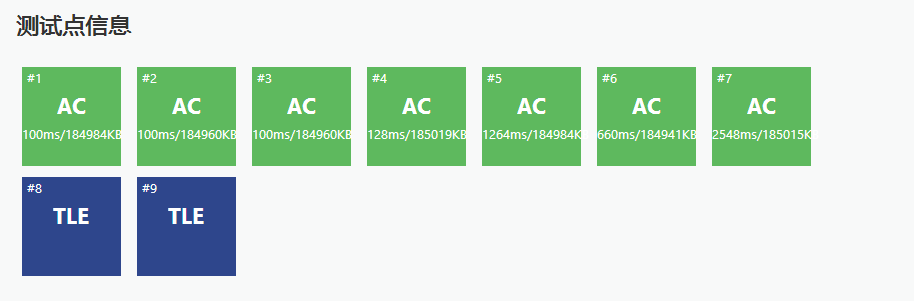递归常数太大，要考虑优化…

FFTの优化——迭代版FFT
这个图也是盗的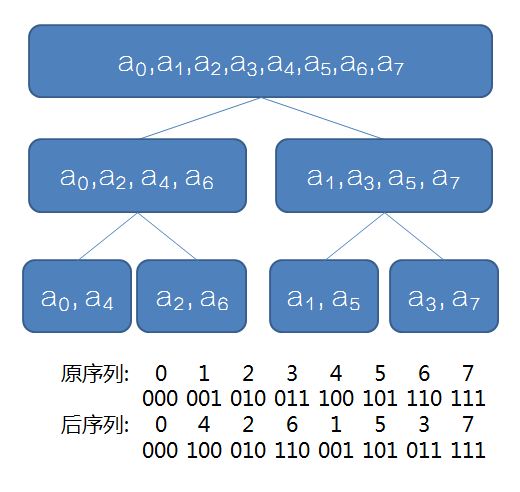这个很容易发现点什么吧？

每个位置分治后的最终位置为其二进制翻转后得到的位置

这样的话我们可以先把原序列变换好，把每个数放在最终的位置上，再一步一步向上合并
一句话就可以$O(n)$预处理第$i$位最终的位置$rev[i]$
fo(i,0,n-1)rev[i]=(rev[i>>1]>>1)|((i&1)<<(bit-1));

至于蝴蝶变换它死了其实是我不会

真·FFT板子
void fft(cp *a,int n,int inv)
{
int bit=0;
while ((1<<bit)<n)bit++;
fo(i,0,n-1)
{
rev[i]=(rev[i>>1]>>1)|((i&1)<<(bit-1));
if (i<rev[i])swap(a[i],a[rev[i]]);//不加这条if会交换两次（就是没交换）
}
for (int mid=1;mid<n;mid*=2)//mid是准备合并序列的长度的二分之一
{
cp temp(cos(pi/mid),inv*sin(pi/mid));//单位根，pi的系数2已经约掉了
for (int i=0;i<n;i+=mid*2)//mid*2是准备合并序列的长度，i是合并到了哪一位
{
cp omega(1,0);
for (int j=0;j<mid;j++,omega*=temp)//只扫左半部分，得到右半部分的答案
{
cp x=a[i+j],y=omega*a[i+j+mid];
a[i+j]=x+y,a[i+j+mid]=x-y;//这个就是蝴蝶变换什么的
}
}
}
}

这个板子好像不是那么好背
至少这个板子已经很优美了

FFT后记
本人版权意识薄弱……

本博客部分知识学习于

https://www.cnblogs.com/RabbitHu/p/FFT.html

https://www.cnblogs.com/zwfymqz/p/8244902.html?mType=Group#_label3

https://blog.csdn.net/ggn_2015/article/details/68922404

$NTT$我来了


展开全文FFT
• 这是快速傅里叶变换的资料，可以通过该资料学习快速傅里叶变换
• 精通matlab傅里叶变换及逆变换和快速傅里叶变换matlab matlab安装教程 matlab下载 matlab图像处理
• 前言 昨天学了一晚上，终于搞懂了FFT。希望能写一篇清楚易懂的题解分享给大家，也进一步加深自己的...FFT（Fast Fourier Transformation），中文名快速傅里叶变换，是离散傅氏变换的快速算法，它是根据离散傅氏变...
前言
昨天学了一晚上，终于搞懂了FFT。希望能写一篇清楚易懂的题解分享给大家，也进一步加深自己的理解。
FFT算是数论中比较重要的东西，听起来就很高深的亚子。但其实学会了（哪怕并不能完全理解)，会实现代码，并知道怎么灵活运用 （背板子） 就行。接下来进入正题。
定义
FFT（Fast Fourier Transformation），中文名快速傅里叶变换，是离散傅氏变换的快速算法，它是根据离散傅氏变换的奇、偶、虚、实等特性，对离散傅立叶变换的算法进行改进获得的。
而在信奥中，一般用来加速多项式乘法。
朴素高精度乘法的时间为$O(n^2)$，但FFT能将时间复杂度降到 $O(nlog_2n)$
学习FFT之前，需要了解一些有关复数和多项式的知识。
有关知识
多项式的两种表示方法
系数表示法
$F[x]=y=a_0x^0+a_1x^1+a_2x^2+......a_nx^n$
{$a_0,a_1,a_2,...,a_n$} 是这个多项式每一项的系数，所以这是多项式的系数表示法
点值表示法
在函数图像中，$F[x]$这个多项式可以被n个点唯一确定，即代入n个点作为$x$,分别解出对应的 $y$，得到n个式子。把这n条式子联立起来成为一个有n条方程的n元方程组，每一项的系数都可以解出来.（可类比二元一次方程）
也就是说，使用{$(x_0,f[x_0])$,$(x_1,f[x_1])$,…,$(x_n,f[x_n])$}就可以完整描述出这个多项式,这就是 多项式的点值表示法
多项式相乘
设两个多项式分别为$f(x)$,$g(x)$,我们要把这两个多项式相乘 (即求卷积)。
如果用系数表示法:
我们要枚举$f$的每一位的系数与$g$的每一位的系数相乘，多项式乘法时间复杂度$O(n^2)$，这也是我们所熟知的高精度乘法的原理。
如果用点值表示法:
$f[x]$={$(x_0,f[x_0])$,$(x_1,f[x_1])$,…,$(x_n,f[x_n])$}
$g[x]$={$(x_0,g[x_0])$,$(x_1,g[x_1])$,…,$(x_n,g[x_n])$}
$f[x]*g[x]$={$(x_0,f[x_0]*g[x_0])$,$(x_1,f[x_1]*g[x_1])$,…,$(x_n,f[x_n]*g[x_n])$}
我们可以发现，如果两个多项式取相同的$x$，得到不同的$y$值，那么只需要$y$值对应相乘就可以了！
复杂度只有枚举$x$的$O(n)$
那么问题转换为将多项式系数表示法转化成点值表示法。
朴素系数转点值的算法叫DFT（离散傅里叶变换）,优化后为FFT（快速傅里叶变换），点值转系数的算法叫IDFT（离散傅里叶逆变换），优化后为IFFT(快速傅里叶逆变换)。之后我会分别介绍。
卷积
其实不理解卷积也没关系，但这里顺便提一下，可以跳过的
卷积与傅里叶变换有着密切的关系。利用一点性质，即两函数的傅里叶变换的乘积等于它们卷积后的傅里叶变换，能使傅里叶分析中许多问题的处理得到简化。
$F(g(x)*f(x)) = F(g(x))F(f(x))$
其中F表示的是傅里叶变换
复数
高中数学会详细讲解，知道的可以跳过这一部分，没学过也没关系，看以下内容应该能很清楚的理解。
1.定义
数集拓展到实数范围内，仍有些运算无法进行。比如判别式小于0的一元二次方程仍无解，因此将数集再次扩充，达到复数范围。
复数$z$被定义为二元有序实数对$(a,b)$,记为$z=a+bi$,这里$a$和$b$是实数，规定$i$是虚数单位。 ($i^2=-1$  即$i=\sqrt{-1}$)
对于复数$z=a+bi$。实数$a$称为复数z的实部(real part),记作$rez=a$.实数$b$称为复数z的虚部(imaginary part)记作 Imz=b.
当虚部等于零时，这个复数可以视为实数。
即当$b=0$时，$z=a$,这时复数成为实数；当且仅当$a=b=0$时，它是实数0；
当z的虚部不等于零时，实部等于零时，常称z为纯虚数。
即当$a=0$且$b≠0$时，$z=bi$，我们就将其称为纯虚数。
将复数的实部与虚部的平方和的正的平方根的值称为该复数的模，记作$∣z∣$。
即对于复数z=a+bi，它的模为$∣z∣=\sqrt{(a^2+b^2)}$
2.复数的几何意义
直接两张图搞定√ (应该可以一目了然)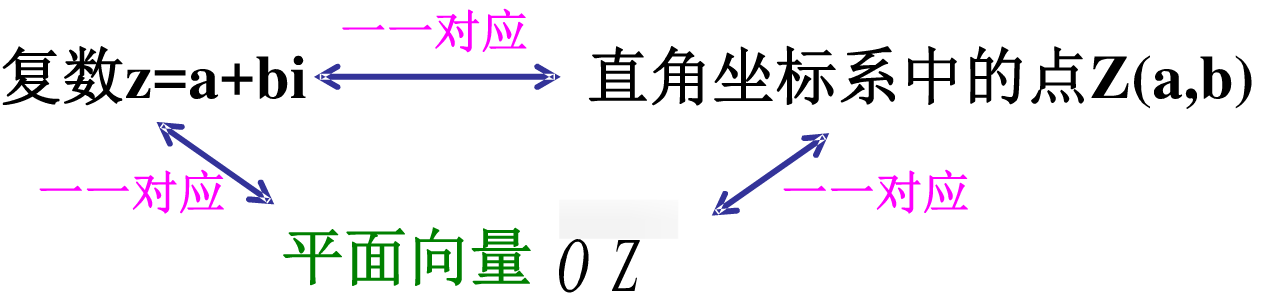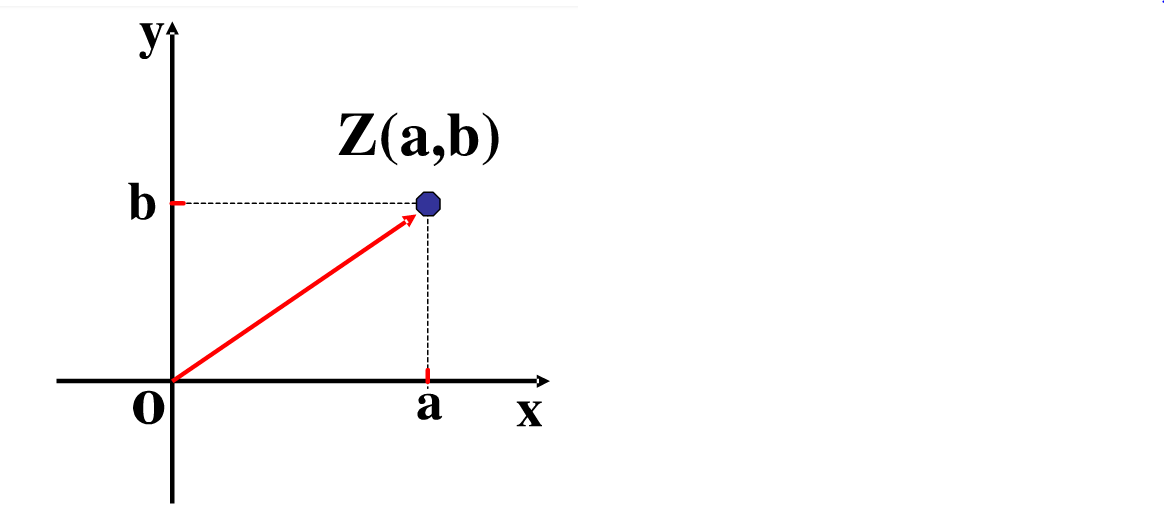3.运算法则
加法法则:$(a+bi)+(c+di)=(a+c)+(b+d)i;$
减法法则:$(a+bi)-(c+di)=(a-c)+(b-d)i;$
注：复数加减满足平行四边形法则：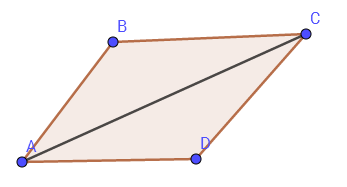乘法法则:$(a+bi)·(c+di)=(ac-bd)+(bc+ad)i$
复数相乘一个重要法则：模长相乘，幅角相加。（这个定理很重要）
模长：这个向量的模长，即这个点到原点的距离。（不懂的可再看下向量的几何意义）。
幅角:  从原点出发、指向x轴正半轴的射线绕原点逆时针旋转至过这个点所经过的角。
在极坐标（可看成平面直角坐标系）下，复数可用模长r与幅角θ表示为$(r,θ)$。对于复数$a+bi$,$r=\sqrt{(a²+b²)}$，$θ=arctan(b/a)$。此时，复数相乘表现为模长相乘，幅角相加。
除法法则：$(a+bi)÷(c+di)=[(ac+bd)/(c²+d²)]+[(bc-ad)/(c²+d²)]i$
4. 共轭复数
一个复数$z=a+bi$的共轭复数为$a−bi$（实部不变，虚部取反），记为 $\overline{z}=a-bi$
当复数模为1时（即|z|=1），与共轭复数互为倒数
证明：$z*\overline{z}=a^2-b^2*i^2=a^2+b^2=|z|^2=1$
FFT加速多项式乘法
由于多项式乘法用点值表示比用系数表示快的多，所以我们先要将系数表示法转化成点值表示法相乘，再将结果的点值表示法转化为系数表示法的过程。
第一个过程叫做FFT（快速傅里叶变换)，第二个过程叫IFFT（快速傅里叶逆变换）
在讲这两个过程之前，首先了解一个概念：
单位根
复数$\omega$满足$\omega^n=1$，称$\omega$是n次单位根
怎么找单位根？
单位圆:圆心为原点、1为半径的圆
把单位圆n等分，取这n个点（或点表示的向量）所表示的复数（即分别以这n个点的横坐标为实部、纵坐标为虚部，所构成的虚数），即为n次单位根。
下图包含了当n=8时，所有的8次单位根，分别记为$\omega_8^1,\omega_8^2.....,\omega_8^8$
(图中圆的半径是1，w表示$\omega$,且下标8已省略）
图是我自己画的，可能有点丑QWQ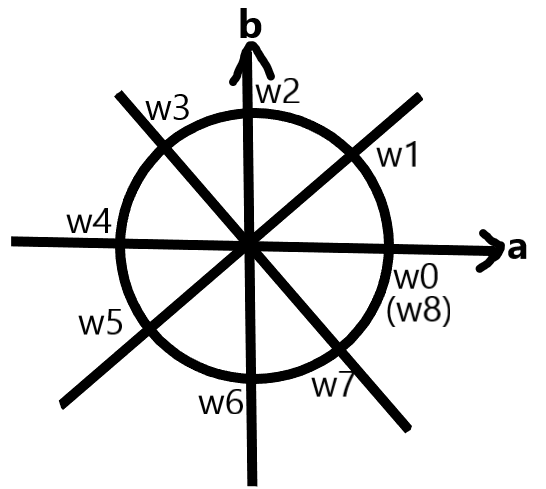由此我们知道如何找单位根啦
从点(1,0)开始（即$\omega_n^1$）,逆时针将这n个点从0开始编号，第k个点对应的虚数记作$\omega_n^k$
由复数相乘法则：模长相乘幅角相加​	可得：
$(\omega_n^1)^k=\omega_n^k$
根据每个复数的幅角，可以计算出所对应的点/向量。$\omega_n^k$ 对应的点/向量是$(cos⁡\frac kn2π,sin⁡\frac kn2π)$，即为复数 $cos⁡\frac kn2π+i *sin⁡\frac kn2π$
单位根的性质
建议记住，因为对之后的分析很重要！！
1.$\omega_n^k=\omega_{2n}^{2k}$
2.$\omega_n^k=-\omega_{n}^{k+\frac n 2}$
3.$\omega_n^0=\omega_{n}^n=1$
至于怎么证明，就是复数相乘时模长相乘幅角相加的原则。或者你直接观察图也可以很显然的得出结论。​
DFT（离散傅里叶变换）
对于任意多项式系数表示转点值表示，例如$F[x]=y=a_0x^0+a_1x^1+a_2x^2+......+a_nx^n$   ，可以随便取任意n个$x$值代入计算,但这样时间复杂度是$O(n^2)$
所以伟大数学家傅里叶取了一些特殊的点代入，从而进行优化。
他规定了点值表示中的$n$个$x$为$n$个模长为1的复数。这$n$个复数不是随机的，而是单位根。
把上述的n个复数（单位根）$\omega_n^0,\omega_n^1.....,\omega_n^{n-1}$代入多项式，能得到一种特殊的点值表示，这种点值表示就叫DFT（离散傅里叶变换）。
FFT（快速傅里叶变换）
虽然DFT能把多项式转换成点值但它仍然是暴力代入$n$个数，复杂度仍然是O(n2)，所以它只是快速傅里叶变换的朴素版。
所以我们要考虑利用单位根的性质，加速我们的运算，得到FFT（快速傅里叶变换）
对于多项式 $A(x)=a_0+a_1x+a_2x^2+...+a_{n−1}x^{n−1}$
将A(x)的每一项按照下标的奇偶分成两部分：
$A(x)=a_0+a_2x^2+...+a_{n−2}x^{n−2}+x*(a_1+a_3x^2+...+a_{n−1}x^{n−2})$
设两个多项式 $A_0(x)$和$A_1(x)$，令：
$A_0(x)=a_0x^0+a_2x^1+...+a_{n-2}x^{n/2-1}$
$A_1(x)=a_1x^0+a_3x^1+...+a_{n-1}x^{n/2-1}$
显然，$A(x)=A_0(x^2)+x*A_1(x^2)$
假设$k，代入$x=ω_n^k$（n次单位根）：
$A(\omega_n^k)$$=A_0(\omega_n^{2k})+\omega_n^{k}*A_1(\omega_n^{2k})$
$=A_0(\omega_\frac n2^{k})+\omega_n^{k}*A_1(\omega_\frac n 2^{k})$
$A(\omega_n^{k+\frac n 2})=A_0(\omega_n^{2k+n})+\omega_n^{k+\frac n 2}*A_1(\omega_n^{2k+n})$
$=A_0(\omega_\frac n2^{k})-\omega_n^{k}*A_1(\omega_\frac n 2^{k})$
考虑A1(x)和A2(x)分别在$(\omega_\frac n 2^{1},\omega_\frac n 2^{2},\omega_\frac n 2^{3},...,\omega_\frac n 2^{\frac n 2-1})$的点值表示已经求出，就可以O(n)求出A(x)在$(\omega_n ^{1},\omega_n ^{2},\omega_n ^{3},...,\omega_n ^{n-1})$处的点值表示。这个操作叫蝴蝶变换
而A1(x)和A2(x)是规模缩小了一半的子问题，所以不断向下递归分治。当n=1的时候返回。
注：这个过程一定要求每层都可以分成两大小相等的部分，所以多项式最高次项一定是2的幂，不是的话直接在最高次项补零QAQ。
时间复杂度$O(nlog_2n)$
IFFT（快速傅里叶逆变换）
我们已经将两个多项式从系数表示法转化成点值表示法相乘后，还要将结果从点值表示法转化为系数表示法，也就是IFFT（快速傅里叶逆变换）
首先思考一个问题，为什么要把$\omega_n^k$（单位根）作为x代入？
当然是因为离散傅里叶变换特殊的性质，而这也和IFFT有关。
一个重要结论
把多项式A(x)的离散傅里叶变换结果作为另一个多项式B(x)的系数，取单位根的倒数即$\omega_n^0,\omega_n^{-1}.....,\omega_n^{1-n}$作为x代入B(x)，得到的每个数再除以n，得到的是A(x)的各项系数，这就实现了傅里叶变换的逆变换了。相当于在FFT基础上再搞一次FFT。
证明（个人觉得写的非常清楚，不想看的跳过吧）~~
设$(y_0,y_1,y_2,...,y_{n−1})$为多项式
$A(x)=a_0+a_1x+a_2x^2+...+a_{n−1}x^{n−1}$的离散傅里叶变换。
设多项式$B(x)=y_0+y_1x+y_2x^2+...+y_{n−1}x^{n−1}$
把离散傅里叶变换的$\omega_n^0,\omega_n^1.....,\omega_n^{n-1}$这n个单位根的倒数，即$\omega_n^0,\omega_n^{-1}.....,\omega_n^{1-n}$作为x代入$B(x)$, 得到一个新的离散傅里叶变换$(z_0,z_1,z_2,...,z{n−1})$
$z_k$=$\sum_{i=0}^{n−1}y_i(ω_n^{-k})^i$
=$\sum_{i=0}^{n−1}(\sum_{j=0}^{n-1}a_j*(\omega_n^i)^j)(ω_n^{-k})^i$
=$\sum_{j=0}^{n-1}a_j*(\sum_{i=0}^{n−1}(\omega_n^i)^{j-k})$
当$j−k=0$时，$\sum_{i=0}^{n−1}(\omega_n^i)^{j-k}=n$
否则，通过等比数列求和可知：
$\sum_{i=0}^{n−1}(\omega_n^i)^{j-k}$=$\frac{(ω_n^{j−k})^n-1}{ω_n^{j−k}-1}$=$\frac{(ω_n^{n})^{j-k}-1}{ω_n^{j−k}-1}$=$\frac{1-1}{ω_n^{j−k}-1}$=0
（因为$\omega_n^n$=$\omega_n^0$=1）
所以
$z_k$=$n*a_k$
$a_k$=$\frac {z_k} n$ ,得证。
怎么求单位根的倒数呢？
单位根的倒数其实就是它的共轭复数 。不明白的可以看看前面共轭复数的介绍
到现在你已经完全学会FFT了，但写递归还是可能会超时，所以我们需要优化
优化：迭代FFT
在进行FFT时，我们要把各个系数不断分组并放到两侧，一个系数原来的位置和最终的位置的规律如下。
初始位置：$\omega_n^0$ $\omega_n^1$ $\omega_n^2$ $\omega_n^3$ $\omega_n^4$ $\omega_n^5$ $\omega_n^6$ $\omega_n^7$
第一轮后：$\omega_n^0$ $\omega_n^2$ $\omega_n^4$ $\omega_n^6$|$\omega_n^1$ $\omega_n^3$ $\omega_n^5$ $\omega_n^7$
第二轮后：$\omega_n^0$ $\omega_n^4$|$\omega_n^2$ $\omega_n^6$|$\omega_n^1$ $\omega_n^5$|$\omega_n^3$ $\omega_n^7$
第三轮后：$\omega_n^0$|$\omega_n^4$|$\omega_n^2$|$\omega_n^6$|$\omega_n^1$|$\omega_n^5$|$\omega_n^3$|$\omega_n^7$
“|”代表分组界限
把每个位置用二进制表现出来。位置x上的数，最后所在的位置是“x二进制翻转得到的数”，例如4(100）最后到了1（001）5(101)最后不变为5(101)，3(011)最后到了6(110)。
所以我们先把每个数放到最后的位置上，然后不断向上还原，同时求出点值表示就可以啦。
迭代版FFT就比之前的递归版快多了,真√$O(nlog_2n)$绝妙算法
代码实现FFT
下面是本人写的FFT加速高精度乘法的代码（并有详细注释）：
#include<bits/stdc++.h>
using namespace std;
//complex是stl自带的定义复数的容器
typedef complex<double> cp;
#define N 2097153
//pie表示圆周率π
const double pie=acos(-1);
int n;
cp a[N],b[N];
int rev[N],ans[N];
char s1[N],s2[N];
//读入优化
int read(){
int sum=0,f=1;
char ch=getchar();
while(ch>'9'||ch<'0'){if(ch=='-')f=-1;ch=getchar();}
while(ch>='0'&&ch<='9'){sum=(sum<<3)+(sum<<1)+ch-'0';ch=getchar();}
return sum*f;
}
//初始化每个位置最终到达的位置
{
int len=1<<k;
for(int i=0;i<len;i++)
rev[i]=(rev[i>>1]>>1)|((i&1)<<(k-1));
}
//a表示要操作的系数，n表示序列长度
//若flag为1，则表示FFT，为-1则为IFFT(需要求倒数）
void fft(cp *a,int n,int flag){
for(int i=0;i<n;i++)
{
//i小于rev[i]时才交换，防止同一个元素交换两次，回到它原来的位置。
if(i<rev[i])swap(a[i],a[rev[i]]);
}
for(int h=1;h<n;h*=2)//h是准备合并序列的长度的二分之一
{
cp wn=exp(cp(0,flag*pie/h));//求单位根w_n^1
for(int j=0;j<n;j+=h*2)//j表示合并到了哪一位
{
cp w(1,0);
for(int k=j;k<j+h;k++)//只扫左半部分，得到右半部分的答案
{
cp x=a[k];
cp y=w*a[k+h];
a[k]=x+y;  //这两步是蝴蝶变换
a[k+h]=x-y;
w*=wn; //求w_n^k
}
}
}
//判断是否是FFT还是IFFT
if(flag==-1)
for(int i=0;i<n;i++)
a[i]/=n;
}
int main(){
n=read();
scanf("%s%s",s1,s2);
//读入的数的每一位看成多项式的一项，保存在复数的实部
for(int i=0;i<n;i++)a[i]=(double)(s1[n-i-1]-'0');
for(int i=0;i<n;i++)b[i]=(double)(s2[n-i-1]-'0');
//k表示转化成二进制的位数
int k=1,s=2;
while((1<<k)<2*n-1)k++,s<<=1;
init(k);
//FFT 把a的系数表示转化为点值表示
fft(a,s,1);
//FFT 把b的系数表示转化为点值表示
fft(b,s,1);
//FFT 两个多项式的点值表示相乘
for(int i=0;i<s;i++)
a[i]*=b[i];
//IFFT 把这个点值表示转化为系数表示
fft(a,s,-1);
//保存答案的每一位(注意进位）
for(int i=0;i<s;i++)
{
//取实数四舍五入，此时虚数部分应当为0或由于浮点误差接近0
ans[i]+=(int)(a[i].real()+0.5);
ans[i+1]+=ans[i]/10;
ans[i]%=10;
}
while(!ans[s]&&s>-1)s--;
if(s==-1)printf("0");
else
for(int i=s;i>=0;i--)
printf("%d",ans[i]);
return 0;
}

后记
这篇博客写了一天，终于写完了，完结撒花✿✿ヽ(°▽°)ノ✿
FWT我来啦！！！


展开全文...